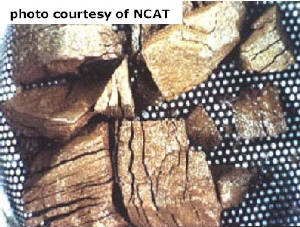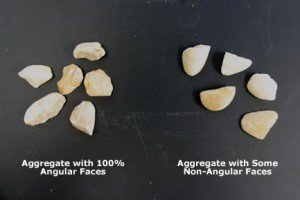# Aggregate Tests

### Sulfate Soundness TestThe soundness test determines an aggregate’s resistance to disintegration by weathering and, in particular, freeze-thaw cycles. Description The most...

### Fine Aggregate Specific GravityOverview The fine aggregate specific gravity test (Figure 1) is used to calculate the specific gravity of a fine...

### Triaxial TestIn a triaxial resilient modulus test a repeated axial cyclic stress of fixed magnitude, load duration and cyclic duration...Overview The gradation and size test (Figure 1) is used to determine aggregate particle size distribution. Size distribution is...

### Fineness ModulusFor aggregates used in PCC, another common gradation description for fine aggregate is the fineness modulus. It is described...

### Particle IndexDescription The particle index test provides a combined shape-texture characterization. This test requires that an aggregate sample be divided...

### Fine Aggregate AngularityOverview The fine aggregate angularity (FAA) test (Figure 1) is an indirect method of assessing the angularity of fine...

### Flat and Elongated ParticlesOverview The flat and elongated particle test is used to determine the dimensional ratios for aggregate particles of specific...

### Percent Fractured Face TestA sample retained on the 4.75 mm (No. 4) sieve is collected and the number of particles with fractured...

### Los Angeles AbrasionOverview The Los Angeles (L.A.) abrasion test (Figure 1) is a common test method used to indicate aggregate toughness...

### Sand EquivalentOverview The sand equivalent test (Figure 1) is a rapid field test to show the relative proportions of fine...

### Coarse Aggregate AngularityOverview The coarse aggregate angularity (CAA) test is a method of determining the angularity of coarse aggregate (Figure 1)....

### Coarse Aggregate Specific GravityOverview The coarse aggregate specific gravity test (Figure 1) is used to calculate the specific gravity of a coarse...